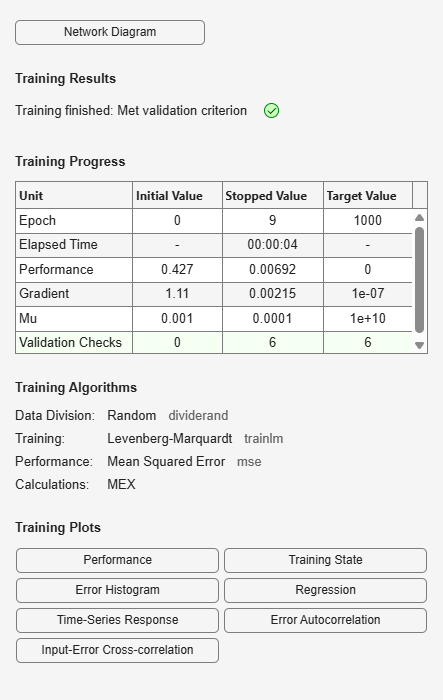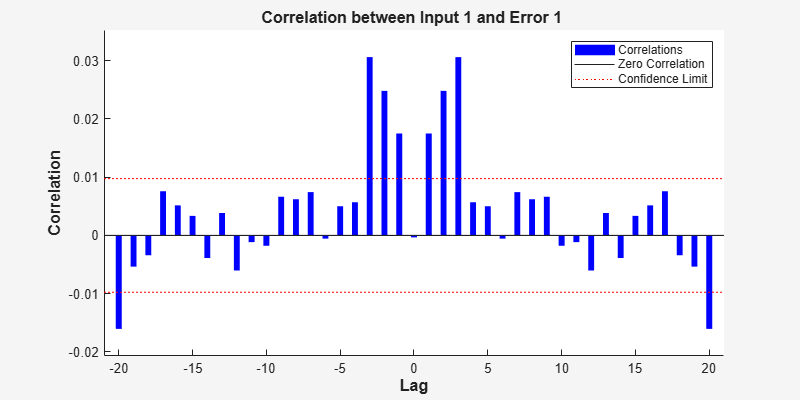# plotinerrcorr

Plot input to error time-series cross-correlation

## Syntax

```plotinerrcorr(x,e) plotinerrcorr(...,'inputIndex',inputIndex) plotinerrcorr(...,'outputIndex',outputIndex) ```

## Description

`plotinerrcorr(x,e)` takes an input time series `x` and an error time series `e`, and plots the cross-correlation of inputs to errors across varying lags.

`plotinerrcorr(...,'inputIndex',inputIndex)` optionally defines which input element is being correlated and plotted. The default is 1.

`plotinerrcorr(...,'outputIndex',outputIndex)` optionally defines which error element is being correlated and plotted. The default is 1.

## Examples

### Plot Cross-Correlation of Inputs to Errors

Here a NARX network is used to solve a time series problem.

```[X,T] = simplenarx_dataset; net = narxnet(1:2,20); [Xs,Xi,Ai,Ts] = preparets(net,X,{},T); net = train(net,Xs,Ts,Xi,Ai);``````Y = net(Xs,Xi,Ai); E = gsubtract(Ts,Y); plotinerrcorr(Xs,E)```## Version History

Introduced in R2010b